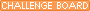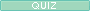molesetc Me Main Page http://www.quia.com/pages/hpedraza.html Send e-mailOUTLINE: Chapter 4: pages 121-135 Review Q's in the book are a good self test. Go To Outline w/ hyperlink notes (good one w/ extra practice & notes!) Avogadro's number: 6.023 E23 Mole: Avogadro's number of anything.      A mole of marbles = 6.023 E23 marbles      A mole of gummy bears = 6.023 E23 gummy bears Molar Mass: (g/mol) Mass of one mole.  Equal to atomic weight but measured in grams. (Get from P.T.) Molecular Weight: Sum of all atomic weights in a molecule. Formula Weight: Same as Molecular Weight (but does not imply molecular (non-metal/non-metal) bonds.     The Reason for The Mole Go To:2 Worlds of Chemistry     You can practice w/ Mole Calculations good examples w/answers and practice!!! molecules<------------->MOLES<-------------------->grams               Av.# / 1 mole         molar mass (from P.T.)               1mole / Av #          g/mole (or 1 mole/?g) ************************************************************ *write down what I give you *look at what I am asking (look at units!) *Use the proper conversion(s) *Take it step by step! ************************************************************ Molarity: Concentration in moles per liter.      If I ask for concentration or molarity, get moles on top and liters on the bottom.  (start w/ what can get moles over one.  Once you have moles, divide by the volume.      ALL OF THE REST OF THE TIME when doing molarity problems, USE the molarity as a conversion factor (moles<-->liters). Percent Composition: (Part) DIVIDED BY (Whole) X 100%      Get atomic masses from the P.T. (parts).  Add them up (don't forget to multiply by the subscripts) to get the whole. Empirical Formula: The LOWEST whole number RATIO of elements in a compound. ***Convert to moles!!!***Use the P.T. If you were given percents, assume 100g.  That way the masses are the same as the percents! Molecular Formula: The actual ration in which elements hook up in a compound. To determine Molecular formula, you need 3 things! Empirical formulas EFM-empirical formula mass MM-molar mass (given in question) Step #1 - MM/EFM = # (a magical whole number) step #2 - multiply the subscripts of the empirical formula by the magic number If you are not given the empirical formula, figure it out! 1>Get Moles of each element!!!!! If I give you grams, great, use the PT to get the atomic mass of each.  24 g C 1 mole = 2 moles C                        12g C 2>Write the wierd Eq (element symbols w/ moles as subscript) 3> Divide ALL subscripts by the smallest. Multiply by 2,3,4 or 5 IF NEEDED. Don't round until the very end!  Only round if it is .9something or .osomething. ARE YOU READY FOR A TEST!?!?!?!? Try links below for more notes and practice.  The practices have answer pages too.
My Quia activities and quizzesMoles "The Beginning"
https://www.quia.com/cb/1824.html
in progressMoles!!!!!: session moles
https://www.quia.com/quiz/599039.html
Will you just type in moles-PLEASE!Too Many Moles!!!!
https://www.quia.com/cb/2102.html
for the test test test test...
 Last updated  2008/09/28 05:23:08 PDT Hits  4404